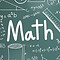top of page

# Mathematics

Mathematics Course and Mathematics Examinations Preparation Course (IGCSE, IB, A-Level, AP, ACT)

Researchers have been studying the ideas and the methods of professional mathematicians and their processes for solving highly complex mathematical problems.  Mathematicians had an intuitive grasp of numbers and the relationships between numbers.  They did not follow the formulas and algorithms taught to elementary students.  They never “carried the two” or cross-multiplied after flipping the denominator or any of the other little tips and tricks that traditional mathematics taught, or if they did, they understood why it worked.

The curriculum developed by Genius Development Workshop helps students learn all the mathematical techniques required in International Mathematical Olympiad and in public examinations, and more importantly, we help students understand why they work.  We teach children multiple ways of attempting to solve a problem and promote strategic critical thinking and creative problem solving.  We encourage children to take initiative or find alternative methods to come to the same right answer.

We incorporate perseverance and critical, strategic reasoning into the standards.  Children at the Genius classroom know that if one way does not work, then perhaps a different method will.  They know that different problems require different approaches.  Our students are prepared for the world as they are able to identify the problem and strategically think through the different tools for problem solving, and then choose the best one.  If that method fails, then they always have backup tools for problem solving.  And they always know how to check their work using different methods.

Here in the Genius classroom, we are helping children reach their full potential, unlocking their imagination and preparing them for a bright future.  The sky is the limit for children in the Genius classroom.

# MATHSThe best way to learn Maths is through a combination of theory and practicals. That is how we teach Maths at Genius Development. Your child will become more curious through understanding the theories and stories behind famous mathematicians taught by Genius’ tutors. Leveraging on this curiosity, we introduce mathematical concepts and practical work to maximise your child’s potential to excel in Maths. Also, we emphasise heavily on the quick applications of mathematics in real-life scenarios. This way, the knowledge learnt will transform your child into a Genius!

A Modular Learning Design for the Seven-Component Curriculum Structure

Mathematics can be a tricky subject, but it does not have to be a hard one.  Concepts of logic and critical thinking learned in math can be applied to many aspects of everyday life.  That was the rationale for the conception and design of the curriculum structure with 7 critical components.

The 7 critical components are

• Arithmetic

• Time and Space

• Algebra

• Geometry

• Number Theory

• Permutations and Combinations

• Applications

01

### Arithmetic

Topics include addition, subtraction, multiplication and division of positive numbers, negative numbers, decimals and fractions.  Simplification of fractions.  Order of operations (BIDMAS).

03

### Algebra

Solving and graphing linear equations, linear inequalities, quadratic equations and quadratic inequalities.  Addition, subtraction, multiplication and division of polynomials.  Factorization.  Exponential functions and logarithmic functions.

05

### Number Theory

Square numbers and perfect cubes.  Prime numbers.  Greatest common factor and least common multiple.  Euclidean Algorithm.  Modular arithmetic.

07

### Applications

Mixture problems.  Problems about rates of work.  Finding the maxima and minima of graphs.  Volume of a solid of revolution.  Area of a surface of revolution.

02

### Time and Space

The concept of distance, speed and time.  Linear motion and curvilinear motion.   Relative velocity between two moving objects.

04

### Geometry

Lines and angles.  Area and volume of plane figures and space figures.  Circle properties.  Congruence and similarity.  Trigonometry.  Polar coordinates.  Polar curves.  Conic sections.

06

### Permutations and Combinations

Counting theory.  Fundamental principle of counting.  Permutations.  Combinations.  Basics of Probability.  Complements and Venn diagrams.

bottom of page